# Non Verbal Reasoning - Figure Matrix

Exercise : Figure Matrix - Section 1
Directions to Solve

In each of the following questions, find out which of the answer figures (1), (2), (3) and (4) completes the figure matrix ?

16.

Select a suitable figure from the four alternatives that would complete the figure matrix.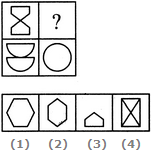1
2
3
4
Explanation:
The two parts of the first figure are rearranged and joined along the longer sides. The common side is then lost to form the second figure.

17.

Select a suitable figure from the four alternatives that would complete the figure matrix.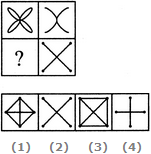1
2
3
4
Explanation:
The second figure is a part of the first figure (but is not exactly the same as the first figure).

18.

Select a suitable figure from the four alternatives that would complete the figure matrix.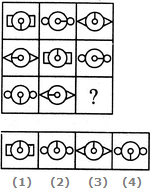1
2
3
4
Explanation:
In each row, the central part of the first figure rotates either 90o CW or 90o ACW to form the central part of the second figure and the central part of the first figure rotates through 180o to form the central part of the third figure. Also, in each row, there are 3 types of side elements - rectangles, circles and triangles.

19.

Select a suitable figure from the four alternatives that would complete the figure matrix.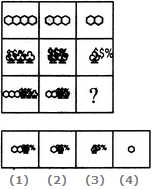1
2
3
4
Explanation:
In each column, the third figure (lowermost figure) contains one less number of hexagons as the first figure (uppermost figure) and the same number of trees as the second figure (middle figure).

20.

Select a suitable figure from the four alternatives that would complete the figure matrix.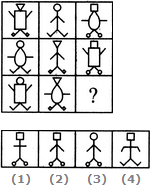1
2
3
4